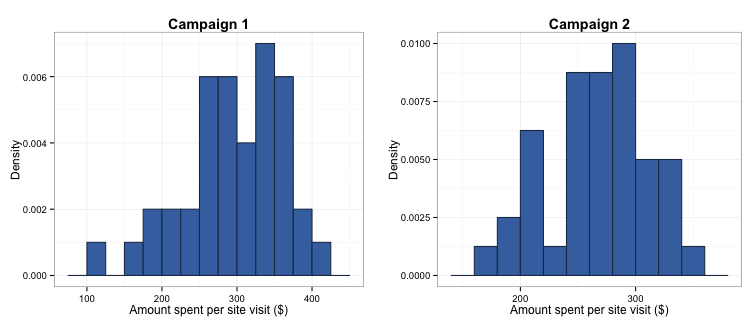# Two-group hypothesis testing: independent samples t-tests

written in

In some of my previous posts, I asked you to imagine that we work for a retail website that sells children’s toys. In the past, they’ve asked us to estimate the mean number of page views per day (see here and here for my posts discussing this problem). Now, they’ve launched two online advertising campaigns, and they want us to see if these campaigns are equally effective or one is better than the other (a technique known as A/B testing or randomised trials).

The way we assess this is a central method in statistical inference called hypothesis testing. The usual workflow of hypothesis testing is as follows:
1. Define your question;
2. Define your hypotheses;
3. Pick the most likely appropriate test distribution (t, Z, binomial, etc.);
4. Compute your test statistic;
5. Work out whether we reject the null hypothesis based whether your test statistic exceeds a critical value under this distribution.

This blog post will through each of these steps in detail, using our advertising campaign problem as an example.

## Defining your question

The first, and most important step to any analysis is working out what you are asking and how you will measure it. A reasonable way to assess whether the advertising campaigns are equally effective or not could be to take all site visits that originate from each of the campaigns and see how much money the company makes from these visits (i.e., the amount of toys the customers who visit buy). A way we could then test if the amount generated differs is to take the mean amount of money made from each campaign and statistically test whether these means are different.

## Defining your hypotheses

The next step is defining hypotheses so we can test these questions statistically. When you define hypotheses, you are trying to compare two possible outcomes. The first is the null hypothesis ($H_0$), which represents the “status quo” and is assumed to be correct until statistical evidence is presented that allows us to reject it. In this case, the null hypothesis is that there is no difference between the mean amount of income generated by each campaign. If we assign $\mu_1$ to be the mean of the first population, and $\mu_2$ to be the mean of the second population, these hypotheses can be stated as:

$$H_0 : \mu_1 = \mu_2$$

or

$$H_0 : \mu_1 - \mu_2 = 0$$

The alternative hypothesis ($H_a$) is that there is a difference between the mean level of income generated by each campaign. More formally, the alternative hypothesis is:

$$H_a : \mu_1 \neq \mu_2$$

or

$$H_a : \mu_1 - \mu_2 \neq 0$$

In other words, we are trying to test whether the difference in the mean levels of income generated by each campaign is sufficiently different from 0 to be meaningful.

## Picking the most appropriate distribution

The most appropriate distribution for our test depends on what we assume the population distribution is. As the next step in our study of which campaign is correct, we take representative samples of site visits originating from each campaign and record how much was purchased (simulated below):

set.seed(567)
campaign.1 <- rt(40, 39) * 60 + 310
campaign.2 <- rt(40, 39) * 58 + 270When we look at the data, it appears close enough to normal. However, our sample is a bit small (40 per campaign), so we should be a bit cautious about using the normal (Z) distribution. Instead, we’ll use a t-distribution, which performs better with “normally-shaped” data that have small sample sizes. According to our samples, the first advertising campaign generated a mean of \$296.42 per visit with a standard deviation of \$65.9, and the second campaign generated a mean of \$267.11 per visit with a standard deviation of \$43.53.

(Technical aside: The reason that the t-distribution performs better than the normal distribution with small samples is because we use the sample standard deviation in our calculation of both the t- and Z-distributions, rather than the true population standard deviation. At large samples, the sample standard deviation is expected to be a very close approximation to the population standard deviation; however, this is not the case in smaller samples. As such, using a Z-distribution for small samples leads to an underestimation of the standard error of the mean and consequently, confidence intervals. Incidently, this also means that as you collect more and more data, the t-distribution behaves more and more like the Z-distribution, meaning that it is a safe bet to use the t-distribution if you are not sure if your sample is big “enough”.)

## Computing your test statistic

The next step is to get some measure of whether these values are different (the test statistic). When talking about hypothesis tests, I pointed out that the null hypothesis can be reframed as $\mu_1 - \mu_2 = 0$, and the alternative hypothesis as $\mu_1 - \mu_2 \neq 0$. As such, we can test our hypotheses by taking the difference of our two campaigns as the difference between the two means.

diff.means <- mean(campaign.1) - mean(campaign.2)


## Take away message

In this post I talked you through a core technique in statistical inference, the two-sample t-test. While this is a very straightforward test to apply in R, choosing when it is an appropriate test to use and whether your data and hypotheses meet the assumptions of this test can be less clear. In addition, the results of a significant test must be interpreted in their practical context. I hope this has given you a starting point for analysing and interpreting the results of A/B testing and similar data.

Finally, the full code used to create the figures in this post is located in this gist on my Github page.